## Hilbert’s simplex distance: A non-separable information monotone distance

October 6, 2021

### Abstract

This note shows that the Hilbert’s metric distance in the probability simplex is a non-separable distance which satisfies the information monotonicity.

Consider the open cone ++d of positive measures (i.e., histograms with d positive bins) with its open probability simplex subset Δd = {(x1,,xd) +d  :   i=1dxi = 1}. A point in Δd represents a multinoulli distribution (categorical distribution).

The f-divergence  between p,q Δd is defined for a convex function f(u) such that f(1) = 0 and f(u) strictly convex at 1 by: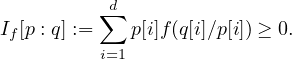For example, the Kullback-Leibler divergence is a f-divergence for f(u) = -log u.

All f-divergences are separable by construction: That is, they can be expressed as sum of coordinate-wise scalar divergences: Here, If[p : q] := i=1dif(p[i] : q[i]), where if is a scalar f-divergence. Moreover, f-divergences are information monotone: That is, let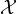= {X1,,Xm} be a partition of {1,,n} into m n pairwise disjoint subsets Xi’s. For p Δn, let p|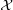Δm denote the induced probability mass function with p|[i] = jXip[i]. Then we have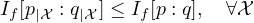Moreover, it can be shown that the only separable divergences satisfying this partition inequality are f-divergences  when n > 2. The special curious binary case n = 2 is dealt in .

Now, consider the non-separable Hilbert distance in the probability simplex :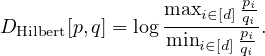This dissimilarity measure is a projective distance on ++d (Hilbert’s projective distance) because we have DHilbert[λp,λq] = DHilbert[p,q] for any λ,λ> 0. However, the Hilbert distance is a metric distance on Δd.

We state the main theorem:

Theorem 1 The Hilbert distance on the probability simplex is an information monotone non-separable distance.

Proof: We can represent the coarse-graining mapping p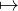p|by a linear application with a m × n matrix A with columns summing up to one (i.e., positive column-stochastic matrix):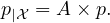For example, the partition= {X1 = {1,2},X2 = {3,4}} (with n = 4 and m = 2) is represented by the matrix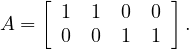Now, a key property of Hilbert distance is Birkhoff’s contraction mapping theorem :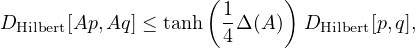where Δ(A) is called the projective diameter of the positive mapping A: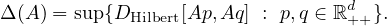Since 0 tanh(x) 1 for x 0, we get the property that Hilbert distance on the probability simplex is an information monotone non-separable distance: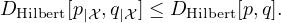Notice that this holds for positive matrices and thus it includes the case of real matrix coefficients encoding deterministic Markov kernels.

Another example of non-separable information monotone distance is Aitchison’s distance on the probability simplex  (using for compositional data analysis).

### References

   Shun-ichi Amari. Information geometry and its applications, volume 194. Springer, 2016.

   Garrett Birkhoff. Extensions of Jentzsch’s theorem. Transactions of the American Mathematical Society, 85(1):219–227, 1957.

   PJ Bushell. On the projective contraction ratio for positive linear mappings. Journal of the London Mathematical Society, 2(2):256–258, 1973.

   Ionas Erb and Nihat Ay. The information-geometric perspective of compositional data analysis. In Advances in Compositional Data Analysis, pages 21–43. Springer, 2021.

   Jiantao Jiao, Thomas A Courtade, Albert No, Kartik Venkat, and Tsachy Weissman. Information measures: the curious case of the binary alphabet. IEEE Transactions on Information Theory, 60(12):7616–7626, 2014.

   Frank Nielsen and Ke Sun. Clustering in Hilbert’s projective geometry: The case studies of the probability simplex and the elliptope of correlation matrices. In Geometric Structures of Information, pages 297–331. Springer, 2019.